#### Thermal Properties, Physics tutorial

Introduction:

The properties considered on the base of atomic viewpoint are specific heat, thermal expansion, equation of state and the thermal conductivity. The most basic approach for the theoretical assessment of such features for a solid is to relate them to the internal energy, the total kinetic energy and potential energy of its constituents.

Definition:

The Specific heat is a measure of the number of degrees of freedom of the oscillating lattice.

Heat capacity:

The heat capacity 'C' is stated as the heat ΔQ which is needed to raise the temperature by ΔT, that is,

C = ΔQ/ΔT

If the procedure is carried out at constant volume V, then ΔQ = ΔE, here ΔE is the increase in internal energy of the system. The heat capacity at constant volume Cv is thus represented by:

Cv = (∂E/∂T)v

The contribution of phonons to the heat capacity of the crystal is termed as the lattice heat capacity.

The net energy of the phonons at temperature 'T' in a crystal can be represented as the sum of the energies over all the phonon modes, in such a way that:

E = Σqp <nqp> (h/2π) ω (q)

Here <nqp> is the thermal equilibrium occupancy of the phonons of wave vector 'q' and mode 'p' (p = 1....3s, here 's' is the number of atoms in a unit cell). The angular brackets represent the average in the thermal equilibrium. Note that we supposed here that the zero-point energy is selected as the origin of the energy, in such a way that the ground energy lies at zero. The average thermal equilibrium can be computed.

Let's take a harmonic oscillator in a thermal bath. The probability to determine this oscillator in an excited state that is characterized through a particular energy En is represented by the Boltzmann distribution:

Pn = Po e{(-n (h/2π)/KBT}

Here the constant P0 is determined from the normalization condition.

n=0Σ Pn = 1

So that,

Po = [n=0Σ e{(-n(h/2π)/KBT}]-1

The average excitation number of the oscillator is represented by:The summation in the numerator can be carried out by using the known property of the geometrical progression:

n=0Σ xn = 1

By using this property we determine:

n=0Σ nxn = x(d/dx)n=0Σ xn = x (d/dx) (1/1-x) (x/(1-x)2)

Here x = e -(h/2π)ω/kT, then we obtain

<n> = x/1-x = 1/x-1-1 = 1/{e(h/2π)/kB) - 1}

The distribution represented by the above equation is termed as the Planck distribution. Coming back to the expression for the net energy of the phonons, we find out that: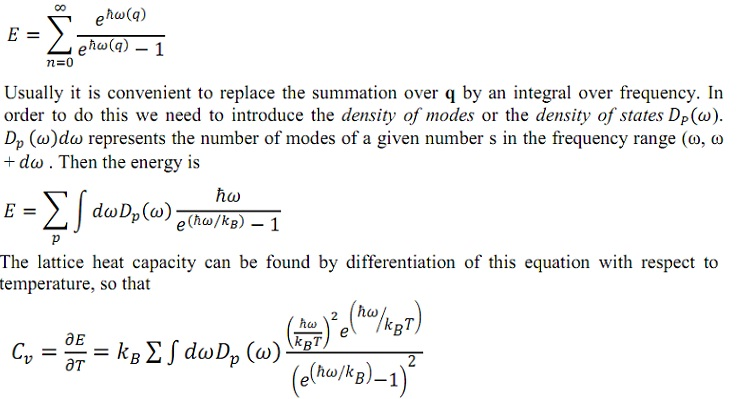Density of state:

Let's take the longitudinal waves in a long bar. The solution for the displacement of atoms is represented by:

u = Aeiqx

Here we omitted a time-dependent factor which is irrelevant for the present illustration. We shall now take the effects of the boundary conditions on this solution. Such boundary conditions are determined through the external constraints applied to the ends of the bar. The most suitable kind of boundary condition is termed as the periodic boundary condition. By this we signify that the right end of the bar is constrained in such a manner that it is for all time in the similar state of oscillation as the left end. It is as if the bar were deformed to a circular shape in such a way that the right end joined the left. Given that the length of the bar is 'L', if we take the origin as being at the left end, the periodic condition signifies that:

u (x = 0) = u (x = L)

From both the above two equation, we obtain:

eiqL = 1

This equation requires a condition on the admissible values of 'q':

q = n (2π/L)

Here n = 0, + 1, ±2 and so on. When such values are plotted all along the q-axis, they make a one-dimensional mesh of regularly spaced points. The spacing among the points is 2π/L. If the bar length is large, the spacing becomes small and the points form a quasi-continuous mesh. Each and every q-value of the equation represents a mode of vibration. Assume that we can select a random interval dq in q-space, and look for the number of modes whose q's lie in this interval. We suppose here that 'L' is large, in such a way that the points are quasi-continuous, that is true for the macroscopic objects. As the spacing between the points is 2π/L, the number of modes is:

(L/2π) dq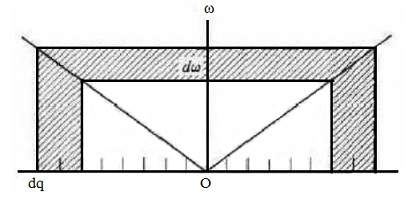We are concerned in the number of modes in the frequency range dω lying between (ω, ω + dω) The density of states D(ω) is stated in such a way that D(ω)dω provides this number.

Comparing this statement by the above equation one might write D(ω)dω = (L/2π) dq, or D(ω) = (L/2π)/(dω/dq). We notice from the figure, though, that in computing D(ω) we should comprise the modes lying in the negative q-region and also in the positive area. The effect is to multiply the above expression for D(ω) via a factor of two. That is,

D(ω) = (L/π)(1/(dω/dq))

We notice that the density of states D(ω) is found out by the dispersion relation:

ω = ω(q)

Now we expand such results to the 3D case. The wave solution analogous to the equation u = Aeiqx is:

u = Aei (qxx + qyy + qzz)

Here the propagation is illustrated by the wave vector q = (qx, qy, qz), whose direction specifies the direction of wave propagation. Here again we require to take into account the boundary conditions. For ease, we suppose a cubic sample whose edge is 'L'. By imposing the periodic boundary conditions, one determines that the allowed values of q should satisfy the condition eiqxL = eiqyL = eiqzL

Thus, the values are represented by:

(qx, qy, qz) = [(l(2π/L), m(2π/L), n(2π/L)]

Here l, m, n are some integers.

If we plot such values in a q-space, as in the figure given below, we get a (3-D) three-dimensional cubic mesh. The volume assigned to each and every point in this q-space is (2π/L)3.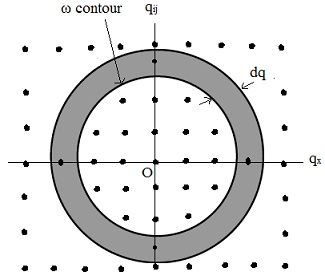Each and every point in the figure above finds out one mode. We now wish for to determine the number of modes lying in the spherical shell between the radii q and q + dq, as represented in figure. The volume of this shell is, 4πq2dq and since the volume per point is (2π/L)3, it follows that the number we search for is:

(L/2π)3 4πq2dq = (V/2π3) 4πq2dq

Here V = L3 is the volume of the sample. By using the definition of the density of modes, this quantity is equivalent to D(ω) dω. Therefore, we arrive at:

D(ω) = (Vq2/2π2)(1/(dω/dq))

We notice that the above equation is valid only for an isotropic solid, in which the vibrational frequency, ω, doesn't base on the direction of q. As well we note that in the above discussion we have related a single mode by each value of q. This is not fairly true for the 3D case, because for each and every 'q' there are in reality three different modes, one longitudinal and two transverse, related by the same value of 'q'. Moreover, in the case of non-Bravais lattice we encompass a few sites, in such a way that the number of modes is 3s, where 's' is the number of non-equivalent atoms. This must be taken into account by index p = 1...3s in the density of states as the dispersion relations for the longitudinal and transverse waves are dissimilar, and acoustic and optical modes are dissimilar.

Debye model:

The Debye model supposes that the acoustic modes provide the dominant contribution to the heat capacity. In the Debye approximation, the velocity of sound is taken a constant independent of polarization as it would be in the classical elastic continuum. The dispersion relation is represented as:

ω = vq

Here 'v' is the velocity of sound.

In this approximation, the density of states is represented by:

D(ω) = Vω2/2π2v3

That is, the density of states rises quadratically by the frequency.

The normalization condition for the density of states finds out the limits of integration over ω. The lower limit is apparently ω = 0. The upper limit can be determine from the condition that the number of vibrational modes in the crystal is finite and is equivalent to the number of degrees of freedom of the lattice. Supposing that there are 'N' unit cells is the crystal, and there is just one atom per cell (in such a way that there are 'N' atoms in the crystal), the total number of phonon modes is 3N. Thus, we can write:

Σp oωD D(ω) dω = 3N

Here the cutoff frequency 'ωD' is termed as the Debye frequency. Supposing that the velocity of the three acoustic modes is independent of the polarization and replacing the above two equation, we get:

ωD = (6π2v3N/V)1/3

The cutoff wave vector that corresponds to this frequency is represented by:

qD = ωD/v = (6π2N/V)1/3

So that the modes of wave vector bigger than qD are not allowed. This is due to the fact that the number of modes having q ≤ qD exhausts the number of degrees of freedom of the lattice.Here a factor of 3 is due to the supposition that the phonon velocity is independent of the polarization. This leads to: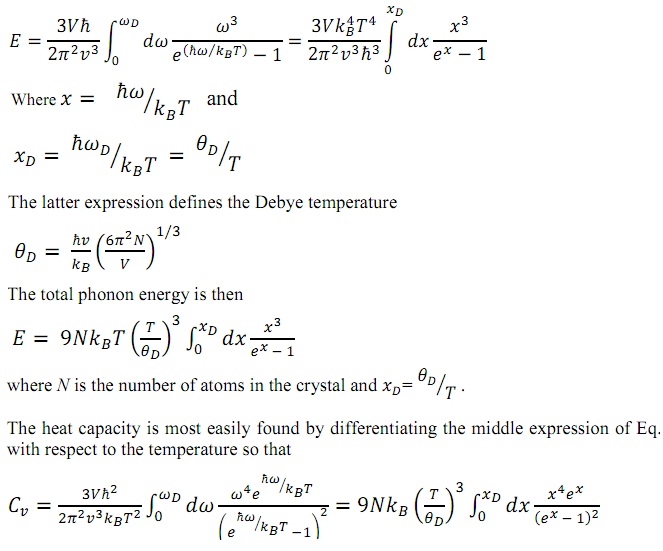In the limit T >> θ, we can expand the expression under the integral and get: Cv = 3NkB. This is precisely the classical value for the heat capacity that is known from the elementary physics. Remember that, according to the elementary thermodynamics the average thermal energy per a degree of freedom is equivalent to E = kBT. Thus for a system of N atoms E = 3NkBT that outcomes in Cv = 3NKB. This is termed as the Dulong-Petit law.

Now consider an opposite limit, that is, T << θ. At very low temperatures we can estimate the total phonon energy by letting the upper limit go to infinity. We get:

E = 9NkBT (T/θD)3 o dx (x3/ex - 1) = 9NkBT (T/θD)3 4/15) = (3π4/5) NkBT (T/θD)3

And thus,

Cv = (12π4/5) NkBT (T/θD)3

We observe that in the Debye model at low temperatures the heat capacity is proportional to T3. The cubic dependence might be understood from the given qualitative argument. At low temperature, just a few modes are excited. These are the modes whose quantum energy (h/2π) ω is less than KBT. The number of such modes might be anticipated by drawing a sphere in the q-space whose frequency ω = (h/2π)/KBT, and counting the number of points inside. This sphere might be termed as the thermal sphere, in analogy by the Debye sphere illustrated above. The number of modes within the thermal sphere is proportional to q3 ≈ ω3 ≈ T3. Each and every mode is completely excited and consists of an average energy equivalent to KBT. Thus the net energy of excitation is proportional to T3 that leads to a specific heat proportional to T3, in agreement by the above equation.

Einstein model:

In the Einstein model the density of states is approximated via a delta function at some frequency ωE that is,

D (ω) = Nδ (ω - ωE)

Here 'N' is the total number of atoms (that is, oscillators). ωE is termed as the Einstein frequency. The thermal energy of the system is then: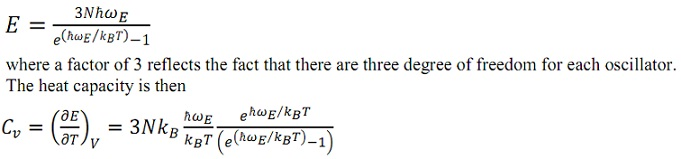The high temperature limit for the Einstein model is similar as that for the Debye model, that is, Cv = 3NkB that is the Dulong-Petit law. The Einstein model is frequently employed to approximate the optical phonon portion of the phonon spectrum.

Thermal conductivity:

If the two ends of a given sample material are at two different temperatures, T1 and T2 (T2 > T1), heat flows down the thermal gradient, that is, from the hotter to the cooler end. Observations represent that the heat current density j (that is, the amount of heat flowing across unit area per unit time) is proportional to the temperature gradient (dT/dx). That is,

j = - K (dT/dx)

The proportionality constant 'K', termed as the thermal conductivity, is a measure of the ease of transmission of heat across the bar (that is, the minus sign is incorporated to make a positive quantity).

Heat might be transmitted in the material through several independent agents. In metals, for illustration, the heat is taken out by both electrons and phonons; however the contribution of the electrons is much larger. In insulators, on the other hand, heat is transmitted wholly via phonons, as there are no mobile electrons in such substances. Here we consider only transmission through phonons.

When we illustrate transmission of heat via phonons, it is suitable to think of these as forming a phonon gas. In each and every region of space there are phonons traveling arbitrarily in all the directions, corresponding to all the q's in the Brillouin zone (BZ), much similar to the molecules in an ordinary gas. The concentration of phonons at the hotter end of the sample is bigger and they move to the cooler end. The benefit of utilizing this gas model is that most of the familiar theories of the kinetic theory of gases can as well be applied here. In specific, thermal conductivity is represented by:

K = (1/3) Cvvl

Here 'Cv' is the specific heat per unit volume, 'v' the velocity of the particle, and 'l' its mean free path. In the present case, 'v' and 'l' refer, obviously, to the velocity and the mean free path of the phonon, correspondingly. The mean free path is stated as the average distances among the two consecutive scattering events, so that l = vτ, here 'τ' is the average time between collisions that is termed as collision time or relaxation time.

Consider a qualitative explanation for the above equation. For ease we take a one-dimensional picture, in which the phonons can move only all along the x-axis. We suppose that a temperature gradient is imposed all along the x-axis. We as well suppose that the collisions between phonons maintain the local thermodynamic equilibrium; in such a way that we can assign local thermal energy density to a specific point of the sample E [T(x)]. The phonons that originate from this point have this energy on average. At a given point x half the phonons come from the high temperature side and half phonons come from the low temperature side. The phonons that arrive to this point from the high-temperature side will, on the average, have had their last collision at point x - l, and will thus carry a thermal energy density of E [T(x - l)]. Their contribution to the thermal current density at point x will thus be the (1/2) vE [T(x - l)]. The phonons arriving at x from the low temperature side, on the other hand, will give - (1/2) vE [T(x + l), as they come from the positive x-direction and are moving in the direction of negative x. Adding such altogether provides:

j = (1/2) vE[T(x - l) + (1/2) vE[T(x + l)

Given that the variation in the temperature over the mean free path is extremely small we might expand this about the point x to determine:

j = vl (dE/dT) (-dT/dx) = v2 τ (dE/dT) (-dT/dx)

This outcome can be simply generalized to the three dimensional case. We require replacing v by the x-component vx, and then average over all the angles. As <vx2> = <vy2> = <vz2> (1/3) v2 and as Cv = dE/dT is the heat capacity we get,

j = (1/3)Cvvl(-dT/dx)

Here 'v' is the phonon velocity.

Tutorsglobe: A way to secure high grade in your curriculum (Online Tutoring)

Expand your confidence, grow study skills and improve your grades.

Since 2009, Tutorsglobe has proactively helped millions of students to get better grades in school, college or university and score well in competitive tests with live, one-on-one online tutoring.

Using an advanced developed tutoring system providing little or no wait time, the students are connected on-demand with a tutor at www.tutorsglobe.com. Students work one-on-one, in real-time with a tutor, communicating and studying using a virtual whiteboard technology.  Scientific and mathematical notation, symbols, geometric figures, graphing and freehand drawing can be rendered quickly and easily in the advanced whiteboard.

Free to know our price and packages for online physics tutoring. Chat with us or submit request at info@tutorsglobe.com